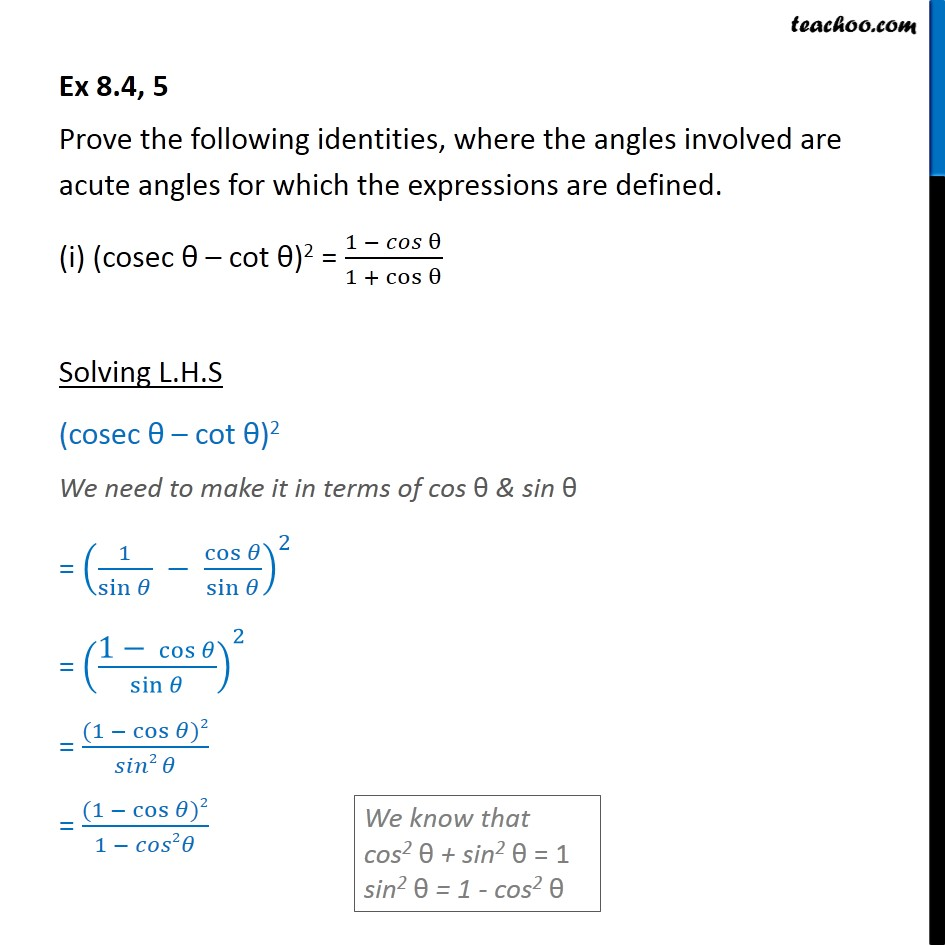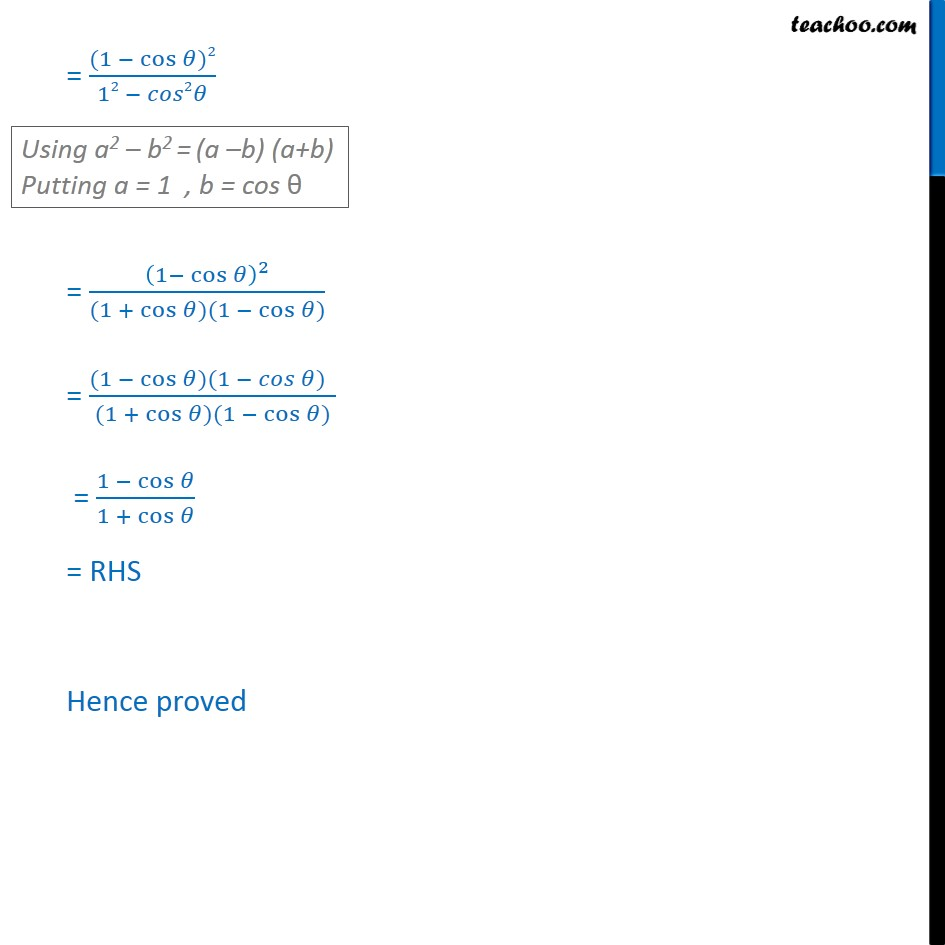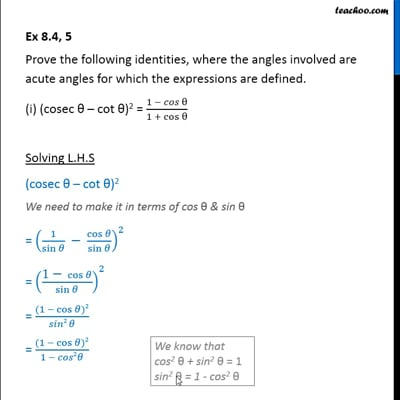Evaluating using Trignometric Identities

Chapter 8 Class 10 Introduction to Trignometry
Concept wiseThis video is only available for Teachoo black users

Introducing your new favourite teacher - Teachoo Black, at only ₹83 per month

### Transcript

Ex 8.4, 5 Prove the following identities, where the angles involved are acute angles for which the expressions are defined. (i) (cosec cot )2 = (1 " " )/(1 + cos ) Solving L.H.S (cosec cot )2 We need to make it in terms of cos & sin = (1/sin cos /sin )^2 = (("1 " cos )/sin )^2 = ((1 cos )2 )/( 2 ) = ((1 cos )2 )/(1 2 ) = ((1 cos )2 )/(12 2 ) = (1 cos )^2/((1 + cos )(1 cos )) = ((1 cos )(1 ) )/((1 + cos )(1 cos )) = (1 cos )/(1 + cos ) = RHS Hence proved